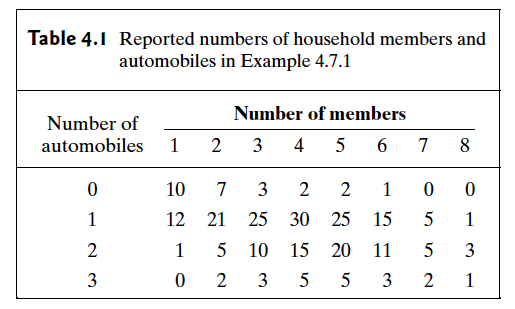Abstract: 本文介绍期望的条件版本，也就是条件期望
Keywords: Expectation，Prediction，Law of Total Probability

# 条件期望

## 条件期望的定义和基本性质 Definition and Basic PropertiesDefinition Conditional Expectation/Mean.Let $X$ and $Y$ be random variables such that the mean of $Y$ exists and is finite.The conditional expectation(or conditional mean) of $Y$ given $X=x$ is denoted by $E(Y|x)$ and is defined to be the expectation of the conditional distribution of $Y$ given $X=x$ .

$$E(Y|x)=\int^{\infty}_{-\infty}yg_2(y|x)dy\text{…………(1)}$$

$$g_2(y|x)=\sum_{\text{ All }y}yg_2(y|x)\text{………………(2)}$$

Definition Conditional Means as Random Variables.Let $h(x)$ stand for the function of $x$ that is denoted $E(Y|x)$ in either (1) or (2).Define the symble $E(Y|X)$ to mean $h(X)$ and call it the conditional mean of $Y$ given $X$

$$E(Y|X=x)=\int^{\infty}_{-\infty}Yf_1(Y|X=x)dy$$

Theorem Law of Total Probability for Expectation.Let $X$ and Y be random variables such that Y has finite mean.Then
$$E[E(Y|X)]=E(Y)$$

\begin{aligned} E[E(Y|X)]&=\int^{\infty}_{-\infty}E(Y|x)f_1(x)dx\\ &=\int^{\infty}_{-\infty}\int^{\infty}_{-\infty}yg_2(y|x)f_1(x)dydx\\ \end{aligned}

$$E[E(Y|X)]=\int^{\infty}_{-\infty}\int^{\infty}_{-\infty}yf(x,y)dydx=E(Y)$$

Let $X$ and $Y$ be random variables,and let $Z=r(X,Y)$ for some function r.The conditional distribution of $Z$ given $X=x$ is the same as the conditional distribution of $r(x,Y)$ given $X=x$

$$E(Z|x)=E(r(x,Y)|x)=\int^{\infty}_{-\infty}r(x,y)g_2(y|x)dy$$

$$E{E[r(X,Y)|X]}=E[r(X,Y)]$$

Defintion Conditional Variance.For every given value $x$ ,let $Var(Y|x)$ denote the variance ofthe conditional distribution of $Y$ given $X=x$ .That is
$$Var(Y|x)=E{[Y-E(Y|x)]^2|x}$$

## 条件期望用来预测 Prediction

Theorem The prediction $d(X)$ that minimizes $E{[Y-d(X)]^2}$ is $d(X)=E(Y|X)$

1. 令 $d(X)=E(Y|X)$ , $d’(X)$ 是一个任意的预测结果，我们只需要证明 $E{[Y-d(X)]^2}\leq E{[Y-d’(X)]^2}$ 成立
2. 根据 $E{E[r(X,Y)|X]}=E[r(X,Y)]$ 有 $Z’=[Y-d’(X)]^2$ 并且 $h’(x)=E(Z’|x)$ 可以把1中的不等式转化成连续函数的期望的形式
$$\int h(x)f_1(x)dx\leq \int h’(x)f_1(x)dx$$
3. 所以我们就把上面要证明的目标转换成了证明 $h(x)\leq h’(x)$
4. 那么我们如果证明了 $E{[Y-d(X)]^2|x}\leq E{[Y-d’(X)]^2|x}$ 就相当于证明了3
5. 假定4中的 $x$ 为常数，根据MSE很容易证明结论

M.S.E. 还和 $Var$ 有关系：当我们预测在给定条件 $X=x$ 的条件下预测 $Y$ 为 $E(Y|x)$ 时其M.S.E就是 $Var(Y|x)$ 如果在 $X$ 未知的情况下最佳预测就是 $E[Y]$

Theorem Law of Total Probability for Variance.If $X$ and $Y$ are arbitrary random variables for which the necessary expectations and variances exist,then $Var(X)=E[Var(Y|X)]+Var[E(Y|X)]$

0%